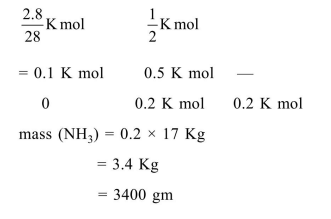# The mass of ammonia in grams produced when 2.8kg

Question:

The mass of ammonia in grams produced when $2.8 \mathrm{~kg}$ of dinitrogen quantitatively reacts with $1 \mathrm{~kg}$ of dihydrogen is_____.

Solution:

$\mathrm{N}_{2}+3 \mathrm{H}_{2} \rightarrow 2 \mathrm{NH}_{3}$